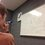# The Golden Ratio: Golden TrianglesHere is the previous post concerning the Golden Ratio. For a collection of all the posts concerning the Golden Ratio, click #GoldenRatio below. A knowledge of trigonometry would be useful in understanding this post.

The last question of the previous post inspired this one. There are certainly more interesting things that result from this pentagon than we would expect. For example, take $\Delta ABF$, or an isoceles triangle with angles $36^{\circ}$, $72^{\circ}$, $72^{\circ}$. Extend the angle bisector from one of the larger angles to the other side. What do you get? If you draw a picture (strongly encouraged) and do some angle chasing, you will find that one of the triangles made is similar to the original triangle! The other resulting triangle should be a familiar $108^{\circ}-36^{\circ}-36^{\circ}$ triangle. Note what happens too when you divide the $108^{\circ}$ angle into $72^{\circ}$ and $36^{\circ}$. What if you trisect the $108^{\circ}$ angle in this triangle? What other dissections can you make? Feel free to post your findings below. The acute triangle is called the golden triangle, while the obtuse triangle is called the golden gnomon.

In my examinations of these figures, I found this especially interesting bit. Let's apply the Law of Cosines on the golden triangle with respect to the $36^{\circ}$ angle:

$1^2 = \phi^2 + \phi^2 - 2 \cdot \phi \cdot \phi \cdot \cos 36^{\circ}$

$\rightarrow 1 = 2\phi^2 (1-\cos 36^{\circ})$

$\rightarrow \cos 36^{\circ} = 1 - \frac{1}{2\phi^2}$

$= 1 - \frac{1}{2 \left( \frac{1 + \sqrt{5}}{2} \right)^2}$

$= 1 - \frac{2}{\left(1 + \sqrt{5}\right)^2}$

$= 1 - \frac{2}{6 + 2\sqrt{5}}$

$= 1 - \frac{1}{3 + \sqrt{5}}$

$= 1 - \frac{3 - \sqrt{5}}{4}$

$= \frac{4 - 3 + \sqrt{5}}{4}$

$= \frac{1}{2} \phi$

Woah! We used to think that sines and cosines of only $30^{\circ}$, $45^{\circ}$, and $60^{\circ}$ (and halves, sums, and multiples thereof) could be expressed nicely. Not any more!

Problem 1: What are the areas of the golden gnomon and golden rhombus? Is there such a thing as a "golden rhombus?" Can you use this information to find the area of a unit pentagon? Post your findings below.

Problem 2: Make a table or list of the sines, cosines, and tangents of some sums and multiples of $36^{\circ}$. Post your findings below.Note by Bob Krueger
7 years ago

This discussion board is a place to discuss our Daily Challenges and the math and science related to those challenges. Explanations are more than just a solution — they should explain the steps and thinking strategies that you used to obtain the solution. Comments should further the discussion of math and science.

When posting on Brilliant:

• Use the emojis to react to an explanation, whether you're congratulating a job well done , or just really confused .
• Ask specific questions about the challenge or the steps in somebody's explanation. Well-posed questions can add a lot to the discussion, but posting "I don't understand!" doesn't help anyone.
• Try to contribute something new to the discussion, whether it is an extension, generalization or other idea related to the challenge.

MarkdownAppears as
*italics* or _italics_ italics
**bold** or __bold__ bold
- bulleted- list
• bulleted
• list
1. numbered2. list
1. numbered
2. list
Note: you must add a full line of space before and after lists for them to show up correctly
paragraph 1paragraph 2

paragraph 1

paragraph 2

[example link](https://brilliant.org)example link
> This is a quote
This is a quote
    # I indented these lines
# 4 spaces, and now they show
# up as a code block.

print "hello world"
# I indented these lines
# 4 spaces, and now they show
# up as a code block.

print "hello world"
MathAppears as
Remember to wrap math in $$ ... $$ or $ ... $ to ensure proper formatting.
2 \times 3 $2 \times 3$
2^{34} $2^{34}$
a_{i-1} $a_{i-1}$
\frac{2}{3} $\frac{2}{3}$
\sqrt{2} $\sqrt{2}$
\sum_{i=1}^3 $\sum_{i=1}^3$
\sin \theta $\sin \theta$
\boxed{123} $\boxed{123}$

Sort by:

Golden gnomon??

- 6 years, 2 months ago